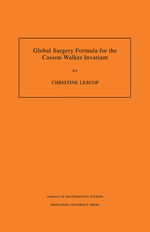# Global Surgery Formula for the Casson-Walker Invariant. (AM-140)

Christine Lescop
Pages: 150
https://www.jstor.org/stable/j.ctt7zv8pj

1. Front Matter
(pp. i-2)
(pp. 3-4)
3. Chapter 1 Introduction and statements of the results
(pp. 5-20)

In 1985, A. Casson defined an integer invariant for oriented integral homology 3-spheres by introducing an appropriate way of counting the conjugacy classes of the SU(2)-representations of their group. He proved that his invariant λcsatisfies the following interesting properties :

λcvanishes on homotopy spheres,

λcchanges sign under orientation reversal,

a simple surgery formula describes the variation of λcunder a surgery on a knot transforming an integral homology sphere into another one, and,

λclifts the Rohlin μ-invariant from$\mathbb{Z}/2\mathbb{Z}$to$\mathbb{Z}$(recall that if M is a$\mathbb{Z}$-homology 3-sphere,...

4. Chapter 2 The Alexander series of a link in a rational homology sphere and some of its properties
(pp. 21-34)

Alexander polynomials are classical invariants in knot theory and have been extensively studied.

The Alexander polynomial of a link in a rational homology sphere can be defined in the powerful and very appropriate context of Reidemeister torsion theory as a Reidemeister torsion of the exterior X of the link (up to a well-determined factor for a knot) and, following [Tu], it can be given a suitable sign, and hence a suitable normalization, if X is equipped with an orientation of${{\text{H}}_{\text{1}}}\text{(X;}\mathbb{R}\text{)}\oplus {{\text{H}}_{\text{2}}}\text{(X;}\mathbb{R}\text{)}$.

The normalization of this Alexander polynomial for oriented links in S3was frrst pointed out by Conway and...

5. Chapter 3 Invariance of the surgery formula under a twist homeomorphism
(pp. 35-59)

This chapter is entirely devoted to the proof of Proposition 3.1.1, that is the invariance of 𝔽Munder the ω-twist described in Definition 1.6.4.

Proposition 3.1.1:(Invariance of𝔽under an ω-twist)

With the notation of Definition1.6.4,${{\mathbb{F}}_{\text{M}}}\text{(}\mathbb{L}\text{)=}{{\mathbb{F}}_{\text{M}}}\text{(}\tilde{\mathbb{L}}\text{)}$

As we observed in the remarks before Equality 1.6.5, the invariance of 𝔽S3under these twist homeomorphisms is sufficient to ensure that 𝔽S3defines an invariant of closed oriented 3-mtnifolds. Proving the invariance of 𝔽Mfor any rational homology sphere M is not more difficult, and it will allow us to consider only generic presentations when when proving the general surgery...

6. Chapter 4 The formula for surgeries starting from rational homology spheres
(pp. 60-80)

§4.2 to §4.5 prove the surgery formula T2 satisfied by λ, that is:

Proposition T2:

For any rational homology sphereM,and for any surgery presentationinM,the surgery formulaF(M,ℍ)is satisfied:$\text{(F(M,}\mathbb{H}\text{)) }\!\!\lambda\!\!\text{ (}{{\text{ }\!\!\chi\!\!\text{ }}_{\text{M}}}\text{(}\mathbb{H}\text{))=}\frac{\left| {{\text{H}}_{\text{1}}}\text{(}{{\text{ }\!\!\chi\!\!\text{ }}_{\text{M}}}\text{(}\mathbb{H}\text{))} \right|}{\left| {{\text{H}}_{\text{1}}}\text{(M)} \right|}\text{ }\!\!\lambda\!\!\text{ (M)+}{{\text{F}}_{\text{M}}}\text{(}\mathbb{H}\text{)}$

Section 4.7 relates the one-component surgery formula to the Walker formula, recalled in §4.6. This relationship implies that λ satisfies Property T5.0:

Property T5.0:

IfM isa rational homology sphere,and ifλwdenotes the Walker invariant as described in[W]:$\text{ }\!\!\lambda\!\!\text{ (M)=}\frac{\left| {{\text{H}}_{\text{1}}}\text{(M)} \right|}{\text{2}}{{\text{ }\!\!\lambda\!\!\text{ }}_{\text{W}}}\text{(M)}$

By definition, Proposition T2 holds for all surgery presentations in S3. Our approach to the proof of Proposition T2 will be to...

7. Chapter 5 The invariant λ for 3-manifolds with nonzero rank
(pp. 81-94)

In this section, we compute λ(M) for any oriented closed 3-manifold M with positive rank. (The rank of a closed 3-manifold M is its first Betti number β1(M).) In order to do this, we frrst give M a surgery presentation as in:

Any oriented closed 3-manifoldMcan be obtained by surgery from a rational homology sphereRaccording to the instructions of a presentation𝕃such that: The linking matrix of𝕃is null and the components of the underlying linkLof𝕃are null-homologous.

The linkLhas thenβ1(M)components, and$\left| {{\text{H}}_{\text{1}}}\text{(R;}\mathbb{Z}\text{)} \right|\text{=}\left| \text{Torsion(}{{\text{H}}_{\text{1}}}\text{(M;}\mathbb{Z}\text{))} \right|$

PROOF: Let β =...

8. Chapter 6 Applications and variants of the surgery formula
(pp. 95-116)

Subsections 6.1 to 6.4 are independent. §6.1 and §6.3 present applications of the surgery formula while §6.2 and §6.4 prove the equivalence between Definition 1.4.8 of the surgery function 𝔽 and the definitions of 𝔽 given in §1.7.

§6.1 is devoted to computing λ for all oriented Seifert fibered spaces using the formula. These spaces are described in [Seif] and [Mo] and by the surgery presentations (taken from [Mo] Fig.12 p.146) of Figures 6.1 and 6.2 below.

The Casson-Walker invariant of Seifert fibered rational homology spheres has already been computed in [L1] (it fortunately gave the same result); and the...

9. Appendix: More about the Alexander series
(pp. 117-146)
10. Bibliography
(pp. 147-148)
11. Index
(pp. 149-151)